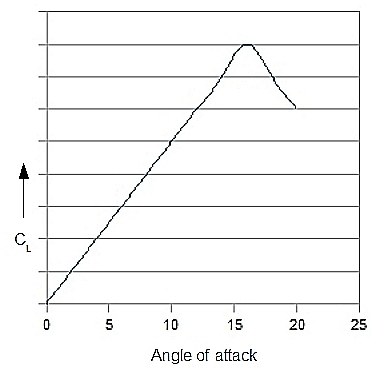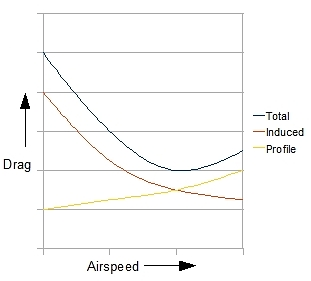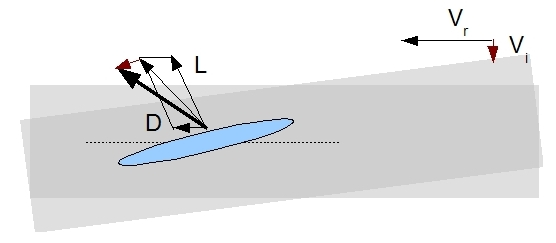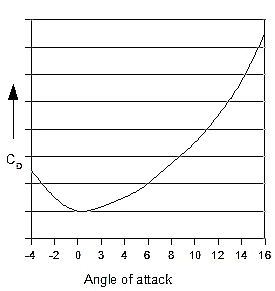# Lift & Drag

## 1 Lift

In this section, we will examine lift and drag in more detail. The force Flift can be calculated from:

Flift = CL (1/2)  ρ V2 S

where:

Flift : Lift (N)
CL : Lift coefficient
ρ: Density of the air
V: True Air Speed or TAS for short (m/s)
S: Airfoil surface area (m2)

In this formula, the coefficient CL depends on the shape and attitude of the airfoil in the airstream. For a symmetrical airfoil with an angle of attack of zero degrees, CL equals zero, meaning that no lift is generated. When the airfoils angle of attack is greater than zero, then the CL value rises accordingly. Angle of attack has a very big impact on the value of CL. The relationship between angle of attack and the lift coefficient is given in the figure bellow. As can be seen, CL rises steep with the angle of attack, until the point where a further increase has a counter productive effect. This is the airfoils stall angle. The encountered loss of lift is due to turbelance and flow separation around the airfoil. So, CL has a maximum value CLmax.An important observation that can be made about the lift formula is that lift depends on velocity squared. Where the rotorblades’ airspeed mainly depends on the speed of angular rotation (RPM), a loss of RPM will inevitably lead to a major loss of lift. That is why maintaining sufficient and constant rotor RPM is one of the key factors when it comes to flying helicopters.

Asymmetrical airfoils are designed to maximise the value CLmax, leading to greater lift. Such an airfoil will also provide lift at a zero angle of attack. Asymmetrical airfoils haven’t been used very often because of the greater forces that come with them. However, by using stronger composite materials, they are also utilised in the design of helicopter rotorblades.

## 2 Drag

### 2.1 Introduction

In this section, we will again examine an airfoil which moves through an air mass, as well looking at the phenomenon of drag. Drag is a friction force, which means it is a force with a direction that is the opposite of movement: it resists movement. When an airfoil moves through an air mass, we can distinguish three different kinds of drag, all with their own distinct properties. These are:

• Profile drag
• Form drag: this kind of drag arises because of the form of the object. Form drag rises with the square of airspeed.
• Skin drag: arises due to friction between the skin and the air mass.
• Induced drag: this is a force component working in the opposite direction of movement (relative to the air mass), and thus acts as drag. Induced drag only exists when there is induced flow (= flow that solely exists because of the downwards acceleration of airflow by the rotor system).

Form and skin drag rise with the square of airspeed, and when combined they define profile drag. The total drag of an airfoil is built up from both profile drag and induced drag, as shown in the figure below.Form and skin drag are true drag forces. Induced drag, however, is a different story.  It is caused by the effect of induced flow on the direction of the lift vector. See the explanation in the figure belowTwo situations are set out in this figure, one with and one without induced flow. In the latter case, the induced drag is zero, whereas in the former, the RAF angle will increase (increase of inflow angle); that is, its direction is moving in a way that is almost parallel to the airfoil. This makes the lift vector point backwards more (relative to the direction of rotor blade movement), and introduces a horizontal force component which is opposite to the rotor movement and thus acts like a resistant force (red arrows in the figure). We call this component induced drag.

When the airspeed component which is parallel to the rotordisc increases (which simply means higher rotor RPM), then the inflow angle will decrease, making the direction of the RAF more parallel to the rotordisc. As a result, the lift vector of the rotorblade will change accordingly: it will point up more and backwards less. Apparently, induced drag decreases rather than increases with airspeed! Indeed, induced drag is not really a friction force.

The total drag of a rotorblade is a function of airspeed. However, rotor RPM must remain fairly constant in helicopters, and drag forces are, therefore, modulated very little by airspeed. However, drag forces are influenced by angle of attack, which is dealt with in the next section.

### 2.2 Drag as a Function of Angle of Attack.

Fdrag = CD (1/2)  ρ V2 S

where:

Fdrag : Drag (N)
CD : Drag coefficient
ρ: Density of the air
V: True Air Speed or TAS for short (m/s)
S: Airfoil surface area (m2)

With their near constant rotor RPM, the most  important way in which rotordrag changes in helicopter operations is due to the change of the angle of attack since this alters the attitude of the airfoil, relative to the air mass. Angle of attack, therefore, has a major influence on the drag coefficient (CD). This relationship is set out in the next figure, which deals with constant airspeed.Note that the drag coefficient never can reach zero (which would mean that there was no drag at all). Of course, the blades are designed to have a low (overall) drag coefficient. At around zero angle of attack, the drag coefficient changes very little, but when the angle of attack increases, it rises rapidly.

Next topic > Lif/Drag Ratio

### Helicopter Theory (Paperback)

• This enormous tome provides comprehensive and detailed coverage of every aspect of helicopter theory. The purpose of the book is to set out for the reader the engineering analyses required to enable the successful design of a rotorcraft. All relevant subjects, such as vertical flight, forward flight, performance, rotating systems, rotary wing dynamics, aerodynamics and aero elasticity, and stability and control are covered. They are explained at an in depth engineering level, always accompanied by accurate mathematical analyses. A good knowledge of mathematics and a degree in engineering are necessary, as these topics are dealt with extensively throughout the text.
• 1089 pages.

### Principles of Helicopter Aerodynamics (Cambridge Aerospace Series) (Hardback)

• Modern treatment of the aerodynamic principles of helicopters and rotating-wing vertical lift aircraft
• Uses mathematics throughout the text
• Concepts are derived from basic enginering principals
• 864 pages

ItemInfoText
1  Chris Chung

Thursday, June 16, 2016 5:22 PMHi, thank you so much for this. I was wondering what does it mean by 'True Airspeed'. I do know that the lift is created by the airfoil where the air above has a faster velocity compared to the air below thus leading to a lower pressure ontop and higher below, which creates the lift. But this 'True airspeed' is this an average of the 2?

Thank You So Much
2  helistartgz

Sunday, June 19, 2016 12:48 PMHi Chris,

No, it is not the average of some other velocities. It is the speed of the air passing the airfoil. For example, when the airspeed is zero, it is the speed at which an airfoil travels.

What can help is to look at a different way of how an airfoils creates lift. Try http://www.helistart.com/Airfoil.aspx

Page: 1

# Do you want to comment this topic?

1: (Book) Cyclic and Collective2: (Book) Principles of Helicopter Flight4: Logitech Extreme 3D Pro Joystick5: Saitek Pro Flight Rudder Pedals